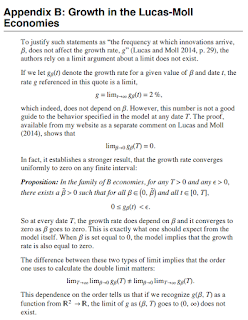## Saturday, May 16, 2015

### The irony of Paul Romer's mathinessFrom Paul Romer's extended mathiness appendix [pdf].

I apparently completely misunderstood Paul Romer's comment about "mathiness". My original interpretation was that Romer was upset about obfuscating political assumptions that aren't substantiated empirically by using fancy math. But then I started reading some of his appendix (pictured above). I was completely wrong.

Paul Romer is upset about the technical rigor of those political assumptions. If the function Lucas and Moll (2014) used allowed you to exchange the order of the limits everything apparently would have been fine! Unfortunately, it turns out that Romer's takedown of Lucas and Moll is the true mathiness.

Lucas and Moll (LM) put forward some economic growth function $g_{\beta}(t)$ where $\beta$ is the "rate of innovation". LM then tell us:

$$\lim_{t \rightarrow \infty} \; g_{\beta}(t) = \text{ 2%}$$

which is independent of $\beta$. Fine. But Romer objects! He shows (somewhere -- couldn't find the comment on LM he was referring to on his website)

$$\lim_{\beta \rightarrow 0} \; g_{\beta}(T) = 0$$

Oh noes! We have a case where the limit depends on the order you take it:

$$\lim_{\beta \rightarrow 0} \; \lim_{T \rightarrow \infty} \; g_{\beta}(T) \neq \lim_{T \rightarrow \infty} \; \lim_{\beta \rightarrow 0} \; g_{\beta}(T)$$

He even goes on to state this in a mathy "proposition" form (pictured above).

Now if $g$ represented the average polarization of an Ising model, this might be interesting (even lending itself to the possibility of a topological solution). But infinity is not readily encountered in a real economy and the situation being described is the idea observing the economy at a date $T$ when the typical time until "knowledge arrives" (I know!) is $1/\beta$. What do these two limits mean in real life?

1. You are observing an economy in which knowledge never arrives ($\beta \rightarrow 0$ first)
2. You are observing an economy after which the knowledge has arrived ($T \rightarrow \infty$ first)

The second one is the sensible limit of Lucas and Moll (2014).

Now what does the time of observation $T$ mean in real life? I am assuming economists don't really pay attention to the big bang or eventual heat death of the universe and that an economy can happen at any time in the lifetime of the universe. That is to say -- economics has a time translation invariance where you could relabel the year 1991 as 10191 and it wouldn't make a bit of difference. Relative times matter, but not absolute times. Which means that I could shift $T$ to any finite, but large value arbitrarily ... in particular I can choose $T \gg 1/\beta$. I can't choose $\beta$ as it represents a real thing: the time between two "knowledge" events.

That is to say there is an existing scale in the model $1/\beta$, the time difference between "knowledge" events. There is no such scale for $T$ unless it is $1/\beta$ and therefore the only sensible limit is:

$$T \gg 1/\beta$$

which is situation 2 above.

Romer is turns out to be ironically wrong because he uses too mathematical a description in a physical theory. You can discard the second first limit as a mathematical curiosity that doesn't represent a real life economy.

I don't have any particular fondness for Lucas, but I think in his zeal to take him down Romer falls for the exact mathiness he purports to dislike!

Update 9 April 2017

I later discussed this more generally. Economists do not appear to understand the physical meaning behind limits, using them only as mathematical procedures.

1.Funny. I wondered about this too when I read the article, although I didn't think of the physics terminology about time-translation invariance, just it depended when you came into the story. I think Romer would argue that there isn't such invariance in that the growth process starts from an initial condition of minimal wealth.

1.You're right, but that's exactly the scale 1/beta (the zero point could only be defined relative to the first knowledge event). Before that point we have no signposts with which to define a scale T.

2.The general consensus seems to be that you had Romer's intent correct originally.

"mathiness", which is restricting your microfoundations in advance to guarantee a particular political result and hiding what you are doing in a blizzard of irrelevant and ungrounded algebra.

1.I'd agree with that in the more general case that DeLong characterizes it correctly, but Romer commits the exact same fault going after Lucas and Moll ... a blizzard of math hiding that what he's doing is really presenting a completely unrealistic scenario as hard headed and empirically grounded economic analysis. I mean, who is living in an economy that never has innovation? It's a bit like saying that there's a limit of string theory where matter never forms and (there is) and saying that limit shows the universe is different from other descriptions ... and therefore the universes with people in them aren't described by the model.

But my original interpretation was a bit different from DeLong's ... I thought it was a general indictment of the complexity and mathematical rigor of models and detachment from empirical reality. Romer's limit above is completely detached from empirical reality. Romer's new post cites the Solow model which as it is taught in modern economics is disconnected from empirical reality.

http://paulromer.net/ed-prescott-is-no-robert-solow-no-gary-becker/
http://informationtransfereconomics.blogspot.com/2015/05/mathiness-and-solow-production-function.html